# Catalog

(Approximate)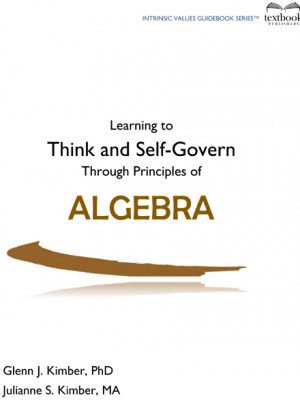Basic Algebra

The intrinsic value for math is “learning to think and self-govern using correct principles.”  This student workbook contains 30 principles of algebra.  An introduction explains the basics of why algebra uses letters to represent unknown numbers.  Ages 12 and up.  (68 pages)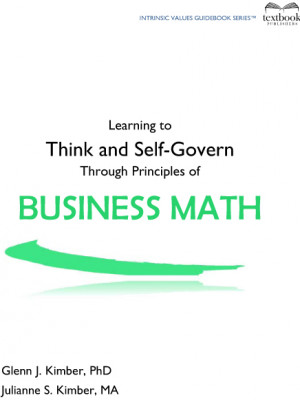The intrinsic value for math is “learning to think and self-govern using correct principles.”  This student workbook contains 37 principles of business math, and an introduction gives an explanation of how “business” works in different scenarios. (53 pages)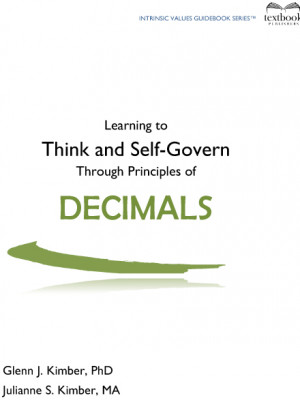Decimals

(Ages 10 and up, 26 pages) The intrinsic value for math is “learning to think and self-govern using correct principles.” This student workbook contains 19 principles of decimals, and an introduction explains the origin of the dollar sign and cents. Writing amounts of money is the most common exposure young … Read more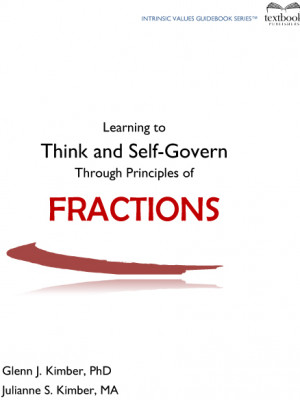Fractions

(Ages 10 and up, 38 pages) The intrinsic value for math is “learning to think and self-govern using correct principles.” This student workbook contains 33 principles of fractions, and students learn the basics of adding, subtracting, multiplying, and dividing fractions, as well as reducing. Vocabulary words students learn along with … Read more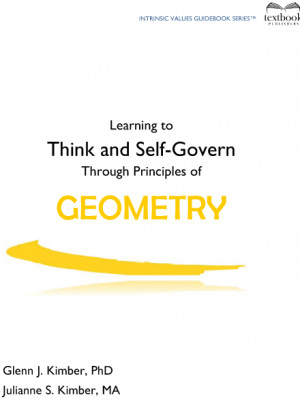Geometry

(Ages 12 and up, 52 pages) The intrinsic value for math is “learning to think and self-govern using correct principles.” This student workbook contains 34 principles of geometry, and an introduction explains the many uses of geometry in occupations and jobs. Students learn the basics of geometrical shapes, as well … Read more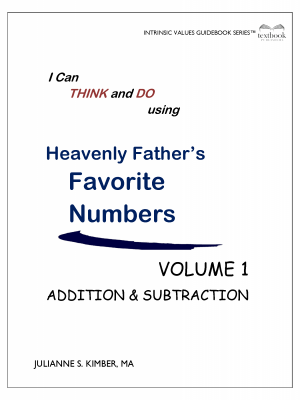Heavenly Father’s Favorite Numbers — Volume 1

After reviewing the basics, students learn the principles of addition and subtraction, including “carrying” and “borrowing.” 96 Pages (Agest 5 – 11)Heavenly Father’s Favorite Numbers — Volume 2

This workbook presents basic principles of multiplication and division.  As with Volume 1, this book will help students understand that everything in the world and in the universe is patterned by God and His use of numbers.  They will practice thinking skills and self-govern with their own creativity.  Timed practice … Read more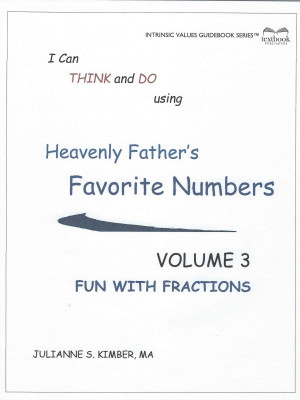Heavenly Father’s Favorite Numbers Volume 3–FRACTIONS

How does Heavenly Father use fractions? You will be surprised to discover many scriptures that refer to fractions — such as a “third” of the hosts of heaven, found in the book of Revelation. With this fun new addition to our junior math program, the younger students will gain a … Read more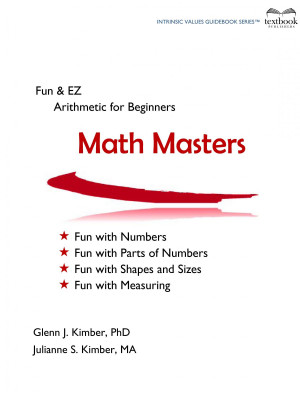Math Masters

Math Masters is written for children ages 4 to 8, with an emphasis on having FUN with numbers and their application.   (109 pages)Numeration

The intrinsic value for math is “learning to think and self-govern using correct principles.” This student workbook contains 20 principles of numeration (whole numbers), and students learn the basics of addition, subtraction, multiplication, and division. (Ages 10 and up, 40 pages)Percents

(Ages 10 and up, 22 pages) The intrinsic value for math is “learning to think and self-govern using correct principles.” This student workbook contains 15 principles of percents, and an introduction explains to the student why it is important to understand how percentages work. Students learn the relationship of percents … Read more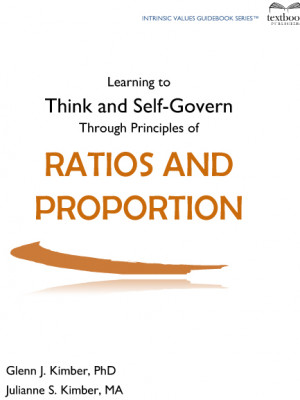Ratios and Proportions

(Ages 10 and up, 21 pages) The intrinsic value for math is “learning to think and self-govern using correct principles.” This student workbook contains 15 principles of ratio & proportion, and an introduction explains the importance of understanding these principles. Students learn various vocabulary words associated with this subject, such … Read more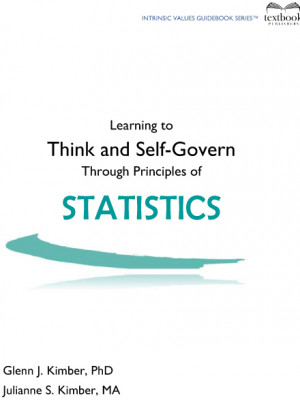Statistics

The intrinsic value for math is “learning to think and self-govern using correct principles.”  This student workbook contains 15 principles of statistics. Students learn the basics of different kinds of charts which compare and analyze data. (26 pages)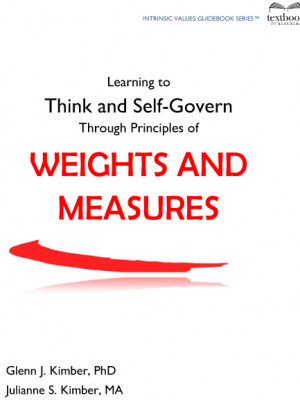Weights and Measures

(Ages 12 and up, 46 pages) The intrinsic value for math is “learning to think and self-govern using correct principles.” This student workbook contains 27 principles of weights and measures. Students learn the basic standards of weighing and measuring with the customary units used in the U.S. They also learn … Read more